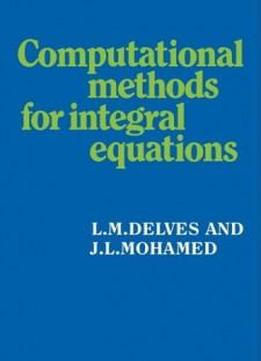Computational Methods For Integral Equations by L. M. Delves / 1988 / English / PDF

Integral equations form an important class of problems, arising frequently in engineering, and in mathematical and scientific analysis. This textbook provides a readable account of techniques for their numerical solution. The authors devote their attention primarily to efficient techniques using high order approximations, taking particular account of situations where singularities are present. The classes of problems which arise frequently in practice, Fredholm of the first and second kind and eigenvalue problems, are dealt with in depth. Volterra equations, although attractive to treat theoretically, arise less often in practical problems and so have been given less emphasis. Some knowledge of numerical methods and linear algebra is assumed, but the book includes introductory sections on numerical quadrature and function space concepts. This book should serve as a valuable text for final year undergraduate or postgraduate courses, and as an introduction or reference work for practising computational mathematicians, scientists and engineers.

Integral equations form an important class of problems, arising frequently in engineering, and in mathematical and scientific analysis. This textbook provides a readable account of techniques for their numerical solution. The authors devote their attention primarily to efficient techniques using high order approximations, taking particular account of situations where singularities are present. The classes of problems which arise frequently in practice, Fredholm of the first and second kind and eigenvalue problems, are dealt with in depth. Volterra equations, although attractive to treat theoretically, arise less often in practical problems and so have been given less emphasis. Some knowledge of numerical methods and linear algebra is assumed, but the book includes introductory sections on numerical quadrature and function space concepts. This book should serve as a valuable text for final year undergraduate or postgraduate courses, and as an introduction or reference work for practising computational mathematicians, scientists and engineers.

views: 718